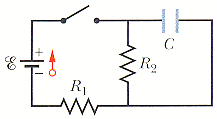# Discharging Capacitor

## Homework Statement

In the figure below, R1 = 5.0 k, R2 = 10.0 k, C = 0.600 µF, and the ideal battery has emf script e = 20.0 V. First, the switch is closed a long time so that the steady state is reached. Then the switch is opened at time t = 0. What is the current in resistor 2 at t = 4.00 ms?## The Attempt at a Solution

I did everything and got .000478. Is this correct? If not, I'll go through all of my steps.

rl.bhat
Homework Helper
Show your steps.